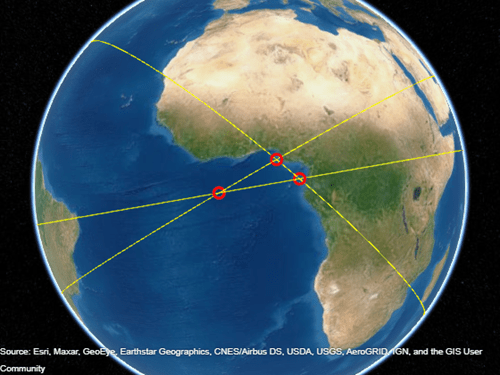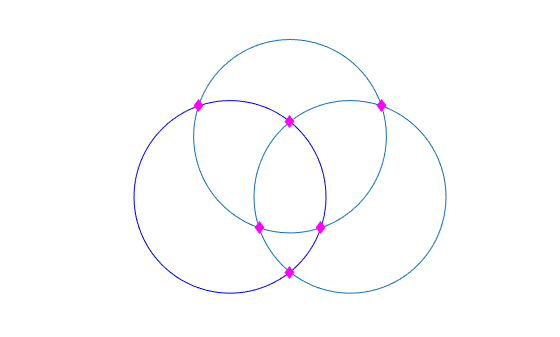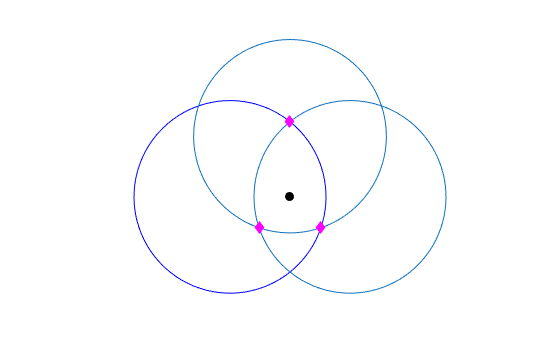# crossfix

Intersection points for pairs of great circles or small circles

## Syntax

``[latIntersect,lonIntersect] = crossfix(lat,lon,az)``
``[latIntersect,lonIntersect] = crossfix(lat,lon,az_radius,ind)``
``[latIntersect,lonIntersect] = crossfix(lat,lon,az_radius,ind,latEstimate,lonEstimate)``
``[latIntersect,lonIntersect] = crossfix(___,units)``
``mat = crossfix(___)``

## Description

example

````[latIntersect,lonIntersect] = crossfix(lat,lon,az)` finds the intersection points of pairs of great circles. Define each great circle by specifying the latitude `lat` and longitude `lon` of a point on the circle and the azimuth `az` at that point.```

example

````[latIntersect,lonIntersect] = crossfix(lat,lon,az_radius,ind)` enables you to find the intersection points of great circles and small circles. When an element of `ind` is `0`, the corresponding elements of `lat` and `lon` define the center of a small circle, and `az_radius` specifies the radius of the small circle. When an element of `ind` is `1`, the corresponding element of `lat` and `lon` define a point on a great circle, and `az_radius` specifies the azimuth.```

example

````[latIntersect,lonIntersect] = crossfix(lat,lon,az_radius,ind,latEstimate,lonEstimate)` finds the intersection point closest to the point specified by `latEstimate` and `lonEstimate`.```
````[latIntersect,lonIntersect] = crossfix(___,units)` specifies the angle units for the coordinates, azimuth, and radii, in addition to any combination of input arguments from the previous syntaxes.```
````mat = crossfix(___)` returns the latitudes and longitudes of the intersection points as the matrix `mat`.```

## Examples

collapse all

Define three great circles by specifying a point on each circle and the azimuth at each point.

```lat = [0 5 0]; lon = [0 5 10]; az = [80 240 310];```

Find the intersections of the great circles.

`[latIntersect,lonIntersect] = crossfix(lat,lon,az)`
```latIntersect = 3×2 0.9022 -0.9022 1.4526 -1.4526 -4.6654 4.6654 ```
```lonIntersect = 3×2 174.8759 -5.1241 8.2683 -171.7317 -175.5811 4.4189 ```

Visualize the results. First, generate the latitude and longitude coordinates of each great circle.

```[lat1,lon1] = track1(lat(1),lon(1),az(1)); [lat2,lon2] = track1(lat(2),lon(2),az(2)); [lat3,lon3] = track1(lat(3),lon(3),az(3));```

Display the great circles on a globe.

```uif = uifigure; g = geoglobe(uif,"Terrain","none"); hold(g,"on") geoplot3(g,lat1,lon1,0,"y") geoplot3(g,lat2,lon2,0,"y") geoplot3(g,lat3,lon3,0,"y")```

Display the intersection points. Note that there are three additional intersection points on the other side of the globe.

```geoplot3(g,reshape(latIntersect,[6 1]),reshape(lonIntersect,[6 1]),0, ... "ro","LineWidth",3)```Define three small circles by specifying their centers and radii. Specify the radii using spherical distances in degrees.

```lat = [0 5 0]; lon = [0 5 10]; r = [8 8 8];```

Find the intersections of the small circles. Indicate that the third argument contains radii (instead of azimuths) by specifying the fourth argument as a vector of `0` values.

```ind = [0 0 0]; [latIntersect,lonIntersect] = crossfix(lat,lon,r,ind)```
```latIntersect = 3×2 7.5594 -2.5744 6.2529 -6.2529 7.5594 -2.5744 ```
```lonIntersect = 3×2 -2.6260 7.5770 5.0000 5.0000 12.6260 2.4230 ```

Visualize the results on a map. First, generate the latitude and longitude coordinates of each small circle.

```[lat1,lon1] = scircle1(lat(1),lon(1),r(1)); [lat2,lon2] = scircle1(lat(2),lon(2),r(2)); [lat3,lon3] = scircle1(lat(3),lon(3),r(3));```

Display the small circles on a map.

```figure axesm("mercator","MapLatLim",[-10 15],"MapLonLim",[-10 20]) axis off geoshow(lat1,lon1,"DisplayType","line","Color","b","LineStyle","-") geoshow(lat2,lon2,"DisplayType","line") geoshow(lat3,lon3,"DisplayType","line")```

Display the intersection points using magenta diamond markers.

```geoshow(reshape(latIntersect,[6 1]),reshape(lonIntersect,[6 1]), ... "DisplayType","point","Marker","d","MarkerEdgeColor","m","MarkerFaceColor","m")```Define three small circles by specifying their centers and radii. Specify the radii using spherical distances in degrees.

```lat = [0 5 0]; lon = [0 5 10]; r = [8 8 8];```

Estimate an intersection point. Then, find the intersections of the small circles that are closest to the estimated point. Indicate that the third argument contains radii (instead of azimuths) by specifying the fourth argument as a vector of `0` values.

```latEstimate = 0; lonEstimate = 5; ind = [0 0 0]; [latIntersect,lonIntersect] = crossfix(lat,lon,r,ind,latEstimate,lonEstimate)```
```latIntersect = 3×1 -2.5744 6.2529 -2.5744 ```
```lonIntersect = 3×1 7.5770 5.0000 2.4230 ```

Visualize the results on a map. First, generate the latitude and longitude coordinates of each small circle.

```[lat1,lon1] = scircle1(lat(1),lon(1),r(1)); [lat2,lon2] = scircle1(lat(2),lon(2),r(2)); [lat3,lon3] = scircle1(lat(3),lon(3),r(3));```

Display the small circles on a map.

```figure axesm("mercator","MapLatLim",[-10 15],"MapLonLim",[-10 20]) axis off geoshow(lat1,lon1,"DisplayType","line","Color","b","LineStyle","-") geoshow(lat2,lon2,"DisplayType","line") geoshow(lat3,lon3,"DisplayType","line")```

Display the estimated point using a black circle marker. Display the intersection points that are closest to the estimated point using magenta diamond markers.

```geoshow(latEstimate,lonEstimate, ... "DisplayType","point","Marker","o","MarkerEdgeColor","k","MarkerFaceColor","k") geoshow(reshape(latIntersect,[3 1]),reshape(lonIntersect,[3 1]), ... "DisplayType","point","Marker","d","MarkerEdgeColor","m","MarkerFaceColor","m")```## Input Arguments

collapse all

Latitudes, specified as an n-element vector, where n is the number of circles. n must be greater than or equal to `2`.

The `crossfix` function interprets each element of `lat` and `lon` depending on the corresponding element of `ind`.

• When an element of `ind` is `1`, the corresponding elements of `lat` and `lon` define a point on a great circle. This is the default behavior.

• When an element of `ind` is `0`, the corresponding elements of `lat` and `lon` define the center of a small circle.

The sizes of `lat`, `lon`, and `az` (or `az_radius` and `ind`) must match.

Data Types: `double`

Longitudes, specified as an n-element vector, where n is the number of circles. n must be greater than or equal to `2`.

The `crossfix` function interprets each element of `lat` and `lon` depending on the corresponding element of `ind`.

• When an element of `ind` is `1`, the corresponding elements of `lat` and `lon` define a point on a great circle. This is the default behavior.

• When an element of `ind` is `0`, the corresponding elements of `lat` and `lon` define the center of a small circle.

The sizes of `lat`, `lon`, and `az` (or `az_radius` and `ind`) must match.

Data Types: `double`

Great circle azimuths, measured clockwise from north, specified as an n-element vector, where n is the number of circles. n must be greater than or equal to `2`.

The sizes of `lat`, `lon`, and `az` must match.

Data Types: `double`

Great circle azimuths or radii of small circles, specified as an n-element vector, where n is the number of circles. n must be greater than or equal to `2`.

The `crossfix` function interprets each element of `az_radius` depending on the corresponding element of `ind`.

• When an element of `ind` is `1`, the corresponding element of `az_radius` is a great circle azimuth. This is the default behavior.

• When an element of `ind` is `0`, the corresponding element of `az_radius` is the radius of a small circle. Specify the radius using a spherical distance in degrees.

The sizes of `lat`, `lon`, `az_radius`, and `ind` must match.

Data Types: `double`

Indicator for azimuths or radii, specified as an n-element vector, where n is the number of circles. n must be greater than or equal to `2`.

The `crossfix` function interprets each element of `lat`, `lon`, and `az_radius` depending on the corresponding element of `ind`.

• To indicate that `lat` and `lon` define a point along a great circle, and that `az_radius` is a great circle azimuth, specify the corresponding element of `ind` as `1`. This is the default behavior.

• To indicate that `lat` and `lon` define the center of a small circle, and that `az_radius` the radius of the small circle, specify the corresponding element of `ind` as `0`.

Data Types: `single` | `double`

Estimated latitude of intersection, specified as a scalar.

When you specify `latEstimate` and the `crossfix` function does not find an intersection, the function issues a warning.

Data Types: `double`

Estimated longitude of intersection, specified as a scalar.

When you specify `lonEstimate` and the `crossfix` function does not find an intersection, the function issues a warning.

Data Types: `double`

Angle unit for the coordinates, specified as one of these options:

• `"degrees"` — Degrees

• `"radians"` — Radians

Data Types: `char` | `string`

## Output Arguments

collapse all

Latitudes of the intersection points, returned as a two-column matrix or a column vector.

• When you do not use `latEstimate` and `lonEstimate` as input arguments, `latIntersect` is a two-column matrix. Each row of the matrix contains the latitude of two intersections.

• When you use `latEstimate` and `lonEstimate` as input arguments, `latIntersect` is a column vector. Each element of the vector contains the latitude of the intersection closest to the estimated point.

The `crossfix` function finds the intersection of each pair of circles. As a result, the number of rows equals `nchoosek(n,2)`, where n is the size of `lat` and `lon`.

When a pair of circles has no intersection, the corresponding row contains `NaN` values.

Longitudes of the intersection points, returned as a two-column matrix or a column vector.

• When you do not use `latEstimate` and `lonEstimate` as input arguments, `lonIntersect` is a two-column matrix. Each row of the matrix contains the longitude of two intersections.

• When you use `latEstimate` and `lonEstimate` as input arguments, `lonIntersect` is a column vector. Each element of the vector contains the longitude of the intersection closest to the estimated point.

The `crossfix` function finds the intersection of each pair of circles. As a result, the number of rows equals `nchoosek(n,2)`, where n is the size of `lat` and `lon`.

When a pair of circles has no intersection, the corresponding row contains `NaN` values.

Latitudes and longitudes of the intersection points, returned as a two-column matrix or a four-column matrix equivalent to `[latIntersect lonIntersect]`.

## Tips

• To find intersection points by using rhumb line azimuths and nautical mile distances, use the `navfix` function.

## Version History

Introduced before R2006a